# Arctic Behavior in Random Tilings

December 8, 1997

Matthew Blum

### Abstract:

A random tiling of a large regular region in the plane often has non-homogeneous behavior throughout the region. Near the center, the tiling appears random, but near the boundaries, the tiling appears uniform and has zero entropy. This paper discusses several different types of tilings and what characterizes their arctic boundaries.

## 1. Introduction

### 1.1 Graphs, Matchings, and Tilings

A bipartite graph is a graph whose vertices can be colored black and red such that each edge connects a black vertex to a red one. Given a bipartite graph that permits a perfect matching, one can think of creating a matching of the graph by pairing every black vertex with a red one. A perfect matching is a collection of edges such that every vertex of the graph belongs to exactly one edge. An example of a graph is shown in figure 1 on the left.

Many regular graphs that permit perfect matchings have interesting properties. One way we can measure a property of a perfect matching is to consider the probability that a given edge is in a random perfect matching. The edge probability of edge E in graph G is the number of perfect matchings of the graph obtained by deleting E and both of its endpoints removed, divided by the number of matchings of G itself.

One of the most fascinating region that has been studied is the Aztec diamond. An Aztec diamond of order n is the union of unit squares in the plane whose centers are contained in the equation |x| + |y| <= n. The graph in figure 1 is an Aztec diamond of order 5. Given a bipartite graph, one can convert the graph into its dual tiling by dimers. A dimer is a shape consisting of two adjacent faces, corresponding to two adjacent vertices in the matching. Each dimer contains two faces where each face corresponds to a vertex in the dual. Figure 1 shows a graph with a perfect matching overlaid on its corresponding tiling on the right.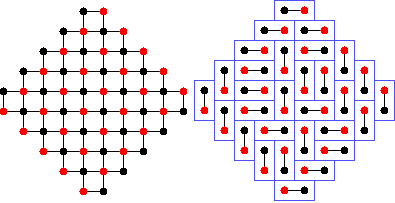Figure 1
Order five Aztec diamond with a matching corresponding to a tiling

It is well known that a random tiling of an Aztec diamond has non-homogeneous behavior, meaning that the probabilities of corresponding edges indicating horizontal or vertical tiles, are not the same if one moves from place to place in a matching. In figure 2, which is a random tiling of an order 50 Aztec diamond, we can see the varying behavior of the edge probabilities.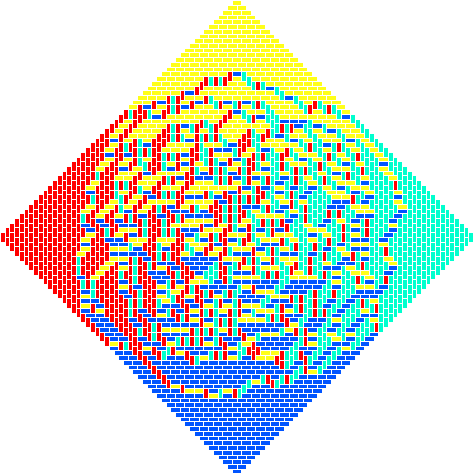Figure 2
Random domino tiling of an order 50 Aztec diamond

### 1.2 Arctic regions

The tiling of the order 50 Aztec diamond as shown in figure 2 has some interesting behavior near the four corners. It appears that the tiling is actually not random at all, but really like all the tiles are fixed in place; they are arranged in a simple periodic pattern. The tiling contains a distinct boundary between the parts that appear random and the part that looks uniform. This boundary is called the Arctic boundary, whose name comes from the fact that everything outside the Arctic boundary appears "frozen" in place. The behavior of the tiling of an Aztec diamond is discussed in much more detail in .

The Aztec diamond is not the only region to have this Arctic phenomenon. It is also true that hexagon tilings with lozenges have this property. Hexagon tilings are discussed in much more detail in . We can see in figure 7, which is a random tiling of an order 20 hexagon, that near the corners, all the tiles appear to be fixed in place as in the Aztec diamond.

### 1.3 Pure phases

It is easy to imagine that given a tiling of a region, one can make another tiling of the region by flip-flopping a small number of tiles. For example on the Aztec diamond graph, it is possible to make another tiling of the Aztec diamond by exchanging two horizontal dominoes for two vertical dominoes given that the two dominoes form a 2x2 block.

If we go back to the dual matching picture, one can look at all the cycles in the graph and consider those cycles that are alternating. A cycle containing vertices {v1, v2, ..., v2n} is alternating if there are exactly n edges between consecutive vertices in the cycle. There are two ways of having an alternating cycle. One way of having an alternating cycle is to have v1, v2 matched; v3, v4 matched, etc. The other way is to have v2, v3 matched; v4, v5 matched, etc.

Thus given a perfect matching of a bipartite graph, we can create another perfect matching, given that there exists an alternating cycle, by replacing the cycle with its opposite. The result will be a different perfect matching. An example of flipping a cycle is shown in figure 3, which shows a 4-cycle being flipped in a domino tiling, and then at the bottom showing how it relates to exchanging two horizontal tiles with two vertical tiles in a 2x2 block.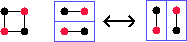Figure 3
A cycle can be matched two ways.

A pure phase of a periodic infinite graph has the property that there are no alternating cycles. We can see in the Aztec diamond picture in figure 2, in the hexagon in figure 7, and in the fortress in figure 10, that near the corners of the tilings, the tiles exist in a pure phase, meaning that there are no local moves that can be made there to produce another tiling.

With dominoes, it is possible to have two different pure phases correspond to the same appearance of tiles. The tiles at the top and the bottom of the Aztec diamond are both horizontal, but they are of different pure phases. Since a domino corresponds to an edge in the corresponding perfect matching, then it is possible to have a horizontal domino with a red vertex on the left, and a different domino with a black vertex on the left. Thus there are four different types of dominoes, two types of verticals and two types of horizontals. Figure 2 shows the four different types of tiles in four different colors.

The Aztec diamond shows four pure phases, and with domino tilings, these are the simplest pure phases. Other pure phases exist, such as herringbone patterns, but these consist of a combination of the simplest pure phases. You can see in figure 4 how a herringbone pattern is just a combination of two simpler pure phases. Define the set of simple pure phases to be the set of pure phases that are independent of each other, so that one phase cannot be created from other pure phases.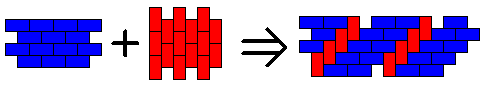Figure 4
Herringbone pattern as a combination of two simple pure phases

It is not true in general, however that an arbitrary periodic lattice will permit a pure phase. Figure 5 shows two counterexamples of periodic semi-regular graphs that do not permit any pure phases.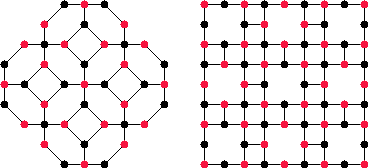Figure 5
Two different lattices that do not permit a pure phase

## 2. Random Tilings

### 2.1 Creating Random Tilings

A random tiling is what one would obtain if he created all the tilings of a region R, put them into a hat, and randomly picked one out of the hat. Thus all the random tilings of R have an equal probability of being chosen.

Except for very small regions, most interesting regions have so many tilings that it would be completely infeasible to try to create all of them, and then randomly choose one. For example, the order 50 Aztec diamond shown above has more tilings than particles in the universe, so it would be impossible to make every tiling and then choose one randomly.

The Propp-Wilson algorithm, as discussed in  is an algorithm for creating a random tiling in a much more efficient way. Since tilings of R exactly correspond to perfect matchings of their dual graphs of R, it is easier to talk about creating a random matching of a bipartite graph G. The Propp-Wilson algorithm involves creating two special matchings and then coupling them together to make a random matching.

Before, we talked about flipping cycles to make different matchings. An alternating cycle can have two states, called the minimum and maximum states. An alternating cycle is in its minimum state if while one looks at the vertices in a clockwise order, then for each edge in the cycle, the black vertex occur before the red one. The cycle is in the maximum state if the red vertex comes before the black vertex. Figure 3 shows a cycle in the graph, along with its minimum and maximum states in a matching. A cycle can be raised or lowered by moving it between its minimum and maximum state. This definition for raising and lowering cycles is arbitrary and the opposite definition is also perfectly equally valid, but it just needs to be consistent.

It is possible now to make a minimum matching of a whole graph simply by flipping all existing alternating cycles to their minimum state so that no maximum alternating cycles still exist. For a finite region, there is always a finite number of lowering moves that need to be made until it is impossible to make any more. It is also true that the minimum matching of any region is unique. The maximum matching is made the same way as the minimum matching, but is made by raising all the cycles until there is no cycle that can be raised. For the Aztec diamond, the minimum matching contains only horizontal tiles and the maximum matching contains only vertical tiles.

The Propp-Wilson algorithm involves making two matchings of the same graph become more like each other until they became the same. During each time step, look at all the cycles in both matchings, and if there is a cycle that is flippable in both matchings in the same location, flip a coin, and make the cycle at the minimum state in both matchings if the coin comes up heads, and maximum otherwise. This way, the matchings become more like each other because a discrepancy at a particular cycle will be removed. Two matchings have coupled if they have become the same as a result of removing discrepancies. If a particular cycle is alternating in only one of the matchings, matchings, then set only that cycle according to the coin flip.

To start the Propp-Wilson algorithm, create the minimum and maximum matchings of the graph. These are the two special matchings that were mentioned before. To make a random matching, we use a "coupling from the past" method that goes as follows: Start at some time -t and evolve the matchings for t time steps. If they have coupled at time zero, then the coupled matching is random. If they have not coupled, then start at time -2t, and run until time zero, but using the same coin flips between -t and 0, as before. If they still have not coupled after 2t time steps, start at time -4t and run for 4t time steps until hitting time zero, but making sure the same coin flips are used between time 2t and 0. We repeat this process, starting from -8t, -16t, and so on as necessary.

It is important to use the "coupling from the past" technique when creating a random tiling, and not to just start with the minimum and maximum at time 0, and evolve them until they couple. Using this alternate technique will result in a biased result and some matchings of G will occur more frequently than other matchings, thus making the random matching not uniformly random.

### 2.2 Vaxrandom

In order to create random matchings of bipartite graphs in practice, we have written computer software to run the Propp-Wilson algorithm. However, just starting with a bipartite graph is not enough to make a random matching. The Propp-Wilson algorithm requires that we have a sample matching of the graph from which we can compute the minimum matching by lowering cycles and the maximum matching by raising cycles.

A newly written program called vaxrandom.c accomplishes all of these things. It first reads in a file representing a graph, and then it runs a max-flow algorithm to either come up with a perfect matching of the graph or demonstrate that the graph does not permit a perfect matching. Once the program has a sample matching, it determines where all of the cycles in the graph are, so that it can raise and lower them. Vaxrandom proceeds by doing as many raising and lowering moves are necessary to make the maximum and minimum matchings. Finally, it runs the Propp-Wilson algorithm by coupling from the past to actually create a random matching.

### 2.3 The Arctic Region

We can define the Arctic region of a matching more precisely now. It is true that it shows a group of tiles where no local raising or lowering moves can be made, but more precisely, the Arctic region of a matching is the largest set of edges of the matching that exactly match the corresponding edges in either the minimum or the maximum matching, that contains no islands in the middle.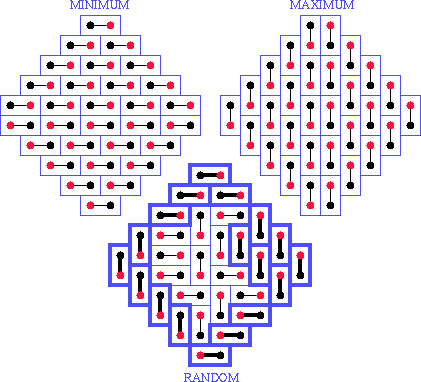Figure 6
Maximum and minimum matchings defining Arctic
region on random matching (same one as in fig 1)

Figure 6 illustrates an order five Aztec diamond matching, along with its minimum and maximum, highlighting the edges that are in the Arctic region.

One property of the Arctic region of a graph that does not contain any forced edges is that it consists of several distinct chunks all touching each other at a single point on the boundary of the region, and the chunks are pieces of the simple pure phases of the graph. We can see in the Aztec diamond that all four pure phases appear with the two from the minimum at the top and bottom and the two from the maximum at the left and right sides.

## 3. What is Known about Arctic Regions

### 3.1 Aztec-like boundaries

It has been proved in  that the shape of the arctic boundary of an Aztec diamond approaches a circle in the limit as the order grows to infinity. It is also true that for a hexagon tiled with lozenges that it also has a circular Arctic region. Figure 7 shows an order 20 hexagon.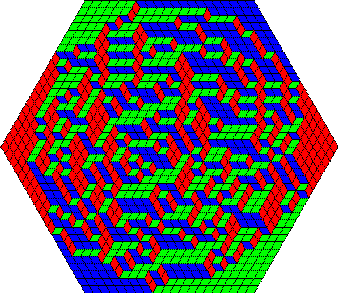Figure 7
Random tiling of an order 20 hexagon

The fact that both Aztec diamonds and hexagons have circular Arctic boundaries suggests that it is possible that other regions should also have Arctic circles. However, some regions do not even have Arctic regions. A domino tiling of a 2n x 2n square has homogeneous behavior everywhere through the tiling showing it is an example of a region with no "frozen" areas. In the limit as n grows to infinity, the probability of seeing any of the four types of dominoes in any location approaches exactly 1/4.

The main question now is when does a region have an Arctic boundary, and if it does, then is the boundary circular? If not, what shape is it?

One important similarity between the Aztec diamond and the hexagon that is not present in the square is that if one traverses the boundary of the matching, the colors of the outermost vertices stay the same for a long stretch, and then change once, but then stay the same again for the next stretch. Figure 1 shows how the Aztec diamond has all black vertices along the upper left and lower right sides, and all red vertices along the upper right and lower left sides. This property is also true of hexagons, but not for squares. We define a boundary having this property an Aztec-like boundary. Along each side of the square, the outermost vertices alternate between red and black and do not stay the same color, so the boundary is not Aztec-like.

One conjecture I present here is that any region with an Aztec-like boundary will illustrate an Arctic region. It is intuitive to see that if a tile near the corner of a region with an Aztec-like boundary is oriented a certain way, then many tiles are forced as a result. Figure 8 shows how this is true for an Aztec diamond and a hexagon. Putting the yellow edge where it is forces the placement of all the blue edges. Because of the large amount of forcing, it would be highly unlikely for an edge equivalent to the yellow one to appear in a random matching.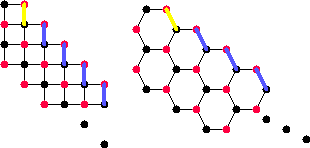Figure 8
How edges get forced along an Aztec-like boundary

### 3.2 Arctic regions of Other Regions

We have seen two examples of regions with circular Arctic regions, but are there other regions with Arctic circles? The square grid and hexagon grid are the only two perfectly periodic graphs that are bipartite. They are bipartite since all of the cycles have an even length. The triangle lattice is the only other perfectly periodic lattice in the plane, but since its cycles are of order 3 and 3 is odd, then one cannot make a bipartite graph from the triangle lattice.

We saw that in both the Aztec diamond and the hexagon that all of the pure phases are present in a random tiling as the order grows larger. A second conjecture I make here is that every region with an Aztec-like boundary will exhibit all of the pure phases of the underlying lattice.

The simplest non-regular lattice with the most regularity in the plane that is bipartite is the square-octagon lattice. One can define a region of the lattice called a fortress that has an Aztec-like boundary. Figure 9 shows an order 5 fortress graph, with its matching and dual tiling overlaid. The fortress has an Aztec-like boundary will all black vertices at the outermost edge on the upper left and lower right sides, and red vertices on the outermost edge on the other two sides.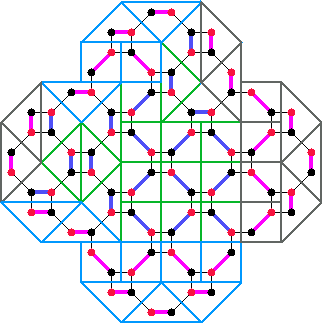Figure 9
Random tiling of an order 5 fortress

Figure 9 shows many things about a fortress tiling. It shows how it is generated from a square-octagon lattice. The blue and purple edges are the edges in the matching, with the purple ones being in the Arctic region. The dimers used to make the tiling are either triangles or squares depending on how they fit in the matching. There are four clumps in the Arctic region as shown by the dimers being accentuated. The clump at the top and the one at the bottom are highlighted in blue tiles and the eastern and western clumps are in gray tiles. The tiles in the middle that are not part of the Arctic region are indicated in green.

Fortress tilings show four pure phases which are all just rotations of each other, as with domino tilings. In fortress tilings, a pure phase consists of distinct rows that alternate between triangles and squares. We can see at the bottom of figure 9 part of a pure phase in blue tiles. Figure 10 shows an order 25 fortress, showing larger Arctic regions. The four chunks are the four different types of pure phases.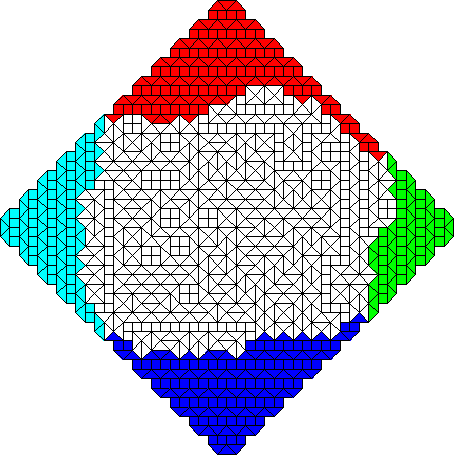Figure 10
An order 25 fortress

## 4. What is Currently Not Known Yet

It is true that the simplest region with an Aztec-like boundary yields an Arctic circle for every grid that is perfectly regular. There are only two examples which are the square and hexagonal grid, and regions on both of these lattices have circular Arctic regions.

It is now possible with vaxrandom to produce random tilings of more general regions so that we can generate large samples of fortresses and other regions to see what the shape of their Arctic regions are. We have shown empirically that fortresses do not have circular Arctic regions. Figure 10 shows an order 25 fortress tiling with its Arctic region accentuated. The four Arctic chunks are highlighted in different colors.

A striking difference between Aztec diamonds and fortresses is the amount of time that is necessary to produce a random tiling. It has been shown experimentally that Aztec diamonds couple in approximately O(n^4) time where n is the order of the Aztec diamond. It is feasible to produce random tilings of large Aztec diamonds of order 100 or greater so that the Arctic boundary is much easier to see. However, fortresses do not appear to couple in O(n^4) time. It is possible that they do not even couple in polynomial time, or if they do, it might be O(n^8) time or longer.

It has not been proven, but it makes sense that the main reason for the long coupling time with fortresses is their large cycles. For the Propp-Wilson algorithm to make two matchings become more like each other, a cycle must be alternating at the same place in both matchings that are coupling. For an octagon, which is an 8-cycle, it is a lot less likely to be alternating than a 4-cycle as in the Aztec diamond. What else is important is that not all the cycles in the fortress grid are of the same length. Half of the cycles in the fortress graph are 8-cycles and the rest are 4-cycles. It is possible that since the 4-cycles are so much more likely to flip than the 8-cycles that the 8-cycles do not get flipped often enough by the Propp-Wilson algorithm.

Since it is infeasible to generate a large random fortress tiling, it is much harder to determine the shape of its Arctic boundary. We can see an approximation in figure 10, though.

It is possible to make many different types of regions with an Aztec-like boundary based on a semi-regular lattice, and all of them yield an Arctic region. The Aztec diamond, hexagon, and fortress are three examples, but there are many others. In fact, I conjecture that the intersection of an Aztec diamond graph and any periodic matching on the square grid, without holes, will produce a region with an Arctic region that converges to an asymptotic shape as the order of the Aztec diamond increases to infinity. One interesting example of this is shown in figure 11. On the left is a picture of a small graph with a simple repeating grid, and on the right is a tiling of an order 30 Aztec diamond tiled with that particular lattice known as the dragon lattice. Note that there are a few forced edges on the matching on the lower left side. We can see here that there are six different pure phases, all highlighted in different colors, and in fact these six pure phases are all six possible pure phases for the dragon lattice.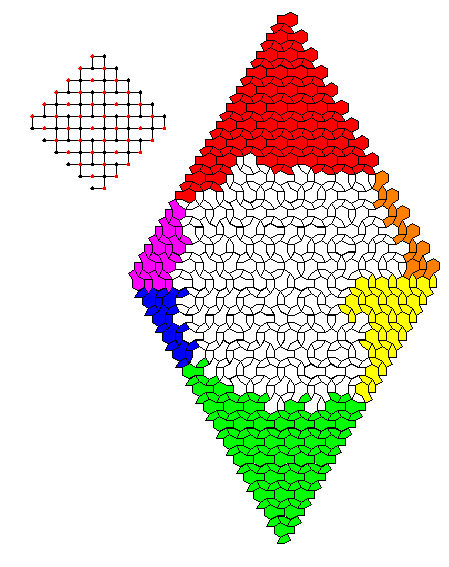Figure 11
An order 30 dragon

## 5. Conclusion

Random tilings show many different and interesting features, including pure phases and Arctic regions. It is striking to see how a random tiling has non-homogeneous behavior and how near the corners of the tiling, the tiles do not appear random at all. We have seen three distinct examples of this behavior.

Even though it is known and has been proved that the shape of the Arctic boundary is a circle for the Aztec diamond and the hexagon, it is not known for more general regions. However, just recently, a method called generalized shuffling has been developed, which allows one to find quickly the probabilities of all of the edges in a graph which is a subset of the Aztec diamond. Generalized shuffling is mentioned in  and  in more detail. Most bipartite graphs can be deformed to fit inside an Aztec diamond, thus allowing us to use the same algorithm to find edge probabilities of more general regions.

The edge probabilities for the Aztec diamond and hexagon have been known for a long time, and the shape of the Arctic region has been known. Now, with the knowledge of the edge probabilities for more general graphs such as the fortress, it may become possible to determine the shape of the fortress Arctic boundary using shuffling.

Shuffling can also be used to find a random perfect matching of a subset of the Aztec diamond graph very quickly. It is described more in . This algorithm is quite valuable in empirically showing the behavior of the Arctic regions of tilings. The slowness of the Propp-Wilson algorithm limits us to fortresses of about order 40, but we can go up to about order 250 for fortresses now with shuffling. The main constraint becomes space instead of time.

Although much information about random tilings is unknown at this time, we are rapidly gaining knowledge about them. A possible next step would be to describe the shape of the Arctic boundary for matchings of much more general regions, and then to possibly describe behavior in the interior of such regions.

## References

 Jockusch, W., Propp, J.,, Shor, P., "Random Domino Tilings and the Arctic Circle Theorem", October 10, 1995.

 Wilson, D., and Propp, J. "How to Get a Perfectly Random Sample From a Generic Markov Chain and Generate a Random Spanning Tree of a Directed Graph", May 21, 1997.

 Cohn, H., Larsen, M., Propp, J., "The Shape of a Typical Boxed Plane Partition", September 2, 1996.

 Propp, J., Wilson, D., "Exact Sampling with Coupled Markov Chains and Applications to Statistical Mechanics", Random Structures and Algorithms. Vol. 9, pp. 223-252, 1996.